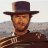# Coding standard deviation based off of historical volatility?

J

#### javin82

##### New member
I need some help programming this indicator. I am trying to plot a 1 standard deviation band above and below a 200 period simple moving average. I want the standard deviation to be based off of historical volatility. Similar to how "the impeccable investor" uses. here is what I have so far:

Code:
``````input HVlength = 20;
def closeLog = Log(close / close);
def SDev = stdev(closeLog,HVlength) * Sqrt(HVlength / (HVlength-1));
def m= SDev * close;
def spike = Average((close - close) / m);

input length = 200;

input numDevDn = -1.0;
input numDevUp = 1.0;
input averageType = AverageType.SIMPLE;

def avg = Average(close, length);
def expDev = ExpAverage(AbsValue(avg - close), length);

def SMADev = SimpleMovingAvg(AbsValue(avg - close), length);
plot UpperBand = avg + numDevUp * Average(SMADev+spike-1, 1);
plot LowerBand = avg + numDevDn * Average(SMADev+spike-1,1);

UpperBand.SetDefaultColor(Color.GREEN);
LowerBand.SetDefaultColor(Color.RED);

thanks for any help in advance!

Last edited by a moderator:#### horserider

##### Well-known member
VIP
Try plotting your defined values to see what you get.

input HVlength = 20;
def closeLog = Log(close / close);
def SDev = stdev(closeLog,HVlength) * Sqrt(HVlength / (HVlength-1));
def m= SDev * close;
def spike = Average((close - close) / m);

input length = 200;

input numDevDn = -1.0;
input numDevUp = 1.0;
input averageType = AverageType.SIMPLE;

def avg = Average(close, length);
def expDev = ExpAverage(AbsValue(avg - close), length);
plot avg1= avg;
plot expDev1 = expdev;

def SMADev = SimpleMovingAvg(AbsValue(avg - close), length);
plot UpperBand = avg + numDevUp * Average(SMADev+spike-1, 1);
plot LowerBand = avg + numDevDn * Average(SMADev+spike-1,1);

UpperBand.SetDefaultColor(Color.GREEN);
LowerBand.SetDefaultColor(Color.RED);

***
You define "def expDev = ExpAverage(AbsValue(avg - close), length);" but seem to never use that value.

J

#### javin82

##### New member
Try plotting your defined values to see what you get.

input HVlength = 20;
def closeLog = Log(close / close);
def SDev = stdev(closeLog,HVlength) * Sqrt(HVlength / (HVlength-1));
def m= SDev * close;
def spike = Average((close - close) / m);

input length = 200;

input numDevDn = -1.0;
input numDevUp = 1.0;
input averageType = AverageType.SIMPLE;

def avg = Average(close, length);
def expDev = ExpAverage(AbsValue(avg - close), length);
plot avg1= avg;
plot expDev1 = expdev;

def SMADev = SimpleMovingAvg(AbsValue(avg - close), length);
plot UpperBand = avg + numDevUp * Average(SMADev+spike-1, 1);
plot LowerBand = avg + numDevDn * Average(SMADev+spike-1,1);

UpperBand.SetDefaultColor(Color.GREEN);
LowerBand.SetDefaultColor(Color.RED);

***
You define "def expDev = ExpAverage(AbsValue(avg - close), length);" but seem to never use that value.

Yes, I have been tweaking this code and must've left that line in from a previous version.
It's not exact, but it is pretty dang close. Thanks for the help!Volatility Cycle Coding Request Questions 0